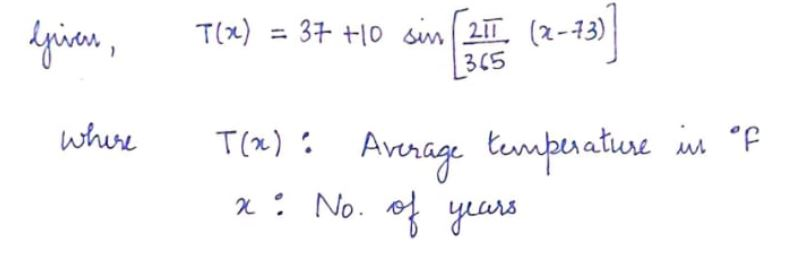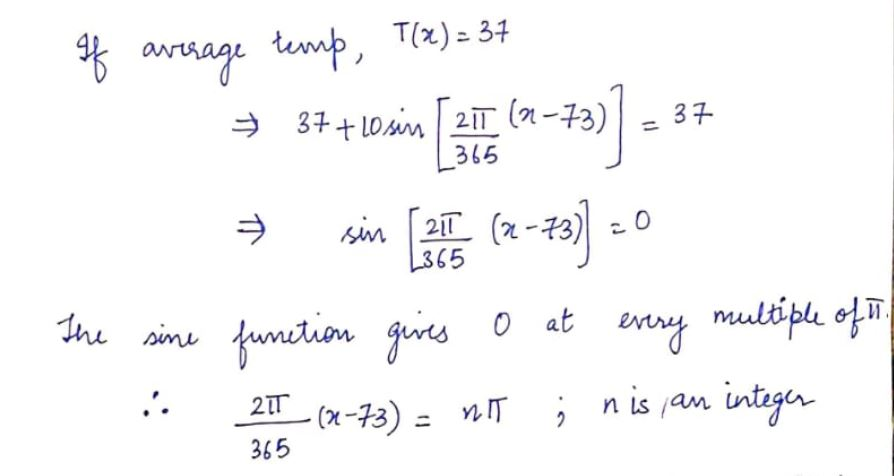# The average temperature  in Dumboldt Valley is given by the following functionT(x)=37 +10 sin((2pi/365)(x-73))Where T is the temperature in degrees Fahrenheit  and x is the number of years.How many years does it take for Dumboldt Valley's average temperature to reach 37 degrees?After reaching 37 degrees the first time, how many years will it take to reach an average temperature of 37 degrees again?

Question
31 views

The average temperature  in Dumboldt Valley is given by the following function

T(x)=37 +10 sin((2pi/365)(x-73))

Where T is the temperature in degrees Fahrenheit  and x is the number of years.

How many years does it take for Dumboldt Valley's average temperature to reach 37 degrees?

After reaching 37 degrees the first time, how many years will it take to reach an average temperature of 37 degrees again?

check_circle

Step 1Step 2...

### Want to see the full answer?

See Solution

#### Want to see this answer and more?

Solutions are written by subject experts who are available 24/7. Questions are typically answered within 1 hour.*

See Solution
*Response times may vary by subject and question.
Tagged in